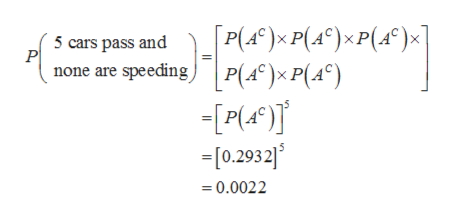# Suppose the distribution of speeds of cars traveling on the I-5 in California is nearly normal with a mean of 72.6 miles/hour and a standard deviation of 4.78 miles/hour. The speed limit on a stretch of the I-5 is 70 miles/hour.(a) What percentage of cars travel above the speed limit?(b) A highway patrol officer is hidden one the side of the freeway. What is the probability that 5 cars pass and none are speeding? Assume that the speeds of cars are independent of each other.(c) On average, how many cars would the highway patrol officer expect to watch until the first car that is speeding?

Question
25 views

Suppose the distribution of speeds of cars traveling on the I-5 in California is nearly normal with a mean of 72.6 miles/hour and a standard deviation of 4.78 miles/hour. The speed limit on a stretch of the I-5 is 70 miles/hour.

(a) What percentage of cars travel above the speed limit?

(b) A highway patrol officer is hidden one the side of the freeway. What is the probability that 5 cars pass and none are speeding? Assume that the speeds of cars are independent of each other.

(c) On average, how many cars would the highway patrol officer expect to watch until the first car that is speeding?

check_circle

Step 1

Solution:

a.The percentage of cars travel above the speed limit is obtained below:

Let the random variable X be the speeds of cars traveling on the I-5 in California follows normal distribution with population mean speed as 72.6 miles/ hour and standard deviation score as 4.78 miles/hour. The speed limit on a stretch of the I-5 is 70 miles/hour.

The percentage of cars travel above the speed limit is 0.7068.

From Excel, 1-NORM.DIST(70,72.6,4.78,TRUE)=0.7068

Step 2

b.The probability that 5 cars pass and none are speeding is obtained below:

Let A be the even that speeding.

P(AC)=1-P(...help_outlineImage TranscriptioncloseP()x P()xP(4f)x] P(4°)x P( °) -P() -[0.2932 5 cars pass and none are speeding, 0.0022 fullscreen

### Want to see the full answer?

See Solution

#### Want to see this answer and more?

Solutions are written by subject experts who are available 24/7. Questions are typically answered within 1 hour.*

See Solution
*Response times may vary by subject and question.
Tagged in

### Other